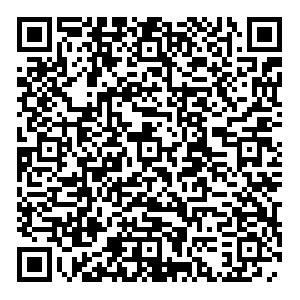# ${{X(2239)}}$and ${{\eta(2225)}}$as hidden-strange molecular states from ${{\Lambda}}{\bar{\Lambda}}$interaction• In this work, we propose the possible assignment of the newly observed $X(2239)$, as well as $\eta(2225)$, as a molecular state from the interaction of a baryon $\Lambda$and an antibaryon $\bar{\Lambda}$. With the help of effective Lagrangians, the $\Lambda\bar{\Lambda}$interaction is described within the one-boson-exchange model with $\eta$, $\eta'$, $\omega$, $\phi$, and $\sigma$exchanges considered. After inserting the potential kernel into the quasipotential Bethe-Salpeter equation, the bound states from the $\Lambda\bar{\Lambda}$interaction can be studied by searching for the pole of the scattering amplitude. Two loosely bound states with spin parities $I^G(J^{PC})=0^+(0^{-+})$and $0^-(1^{--})$appear near the threshold with almost the same parameter. The $0^-(1^{--})$state can be assigned to $X(2239)$observed at BESIII, which is very close to the $\Lambda\bar{\Lambda}$threshold. The scalar meson $\eta(2225)$can be interpreted as a $0^+(0^{-+})$state from the $\Lambda\bar{\Lambda}$interaction. The annihilation effect is also discussed through a coupled-channel calculation plus a phenomenological optical potential. It provides large widths to two bound states produced from the $\Lambda\bar{\Lambda}$interaction. The mass of the $1^-$state is slightly larger than the mass of the $0^-$state after including the annihilation effect, which is consistent with our assignment of these two states as $X(2239)$and $\eta(2225)$, respectively. The results suggest that further investigation is required to understand the structures near the $\Lambda\bar{\Lambda}$threshold, such as $X(2239)$, $\eta(2225)$, and $X(2175)$.
••Get Citation
Jun-Tao Zhu, Yi Liu, Dian-Yong Chen, Longyu Jiang and Jun He. $X(2239)$and $\eta(2225)$as hidden-strange molecular states from $\Lambda\bar{\Lambda}$interaction[J]. Chinese Physics C.
Jun-Tao Zhu, Yi Liu, Dian-Yong Chen, Longyu Jiang and Jun He. $X(2239)$and $\eta(2225)$as hidden-strange molecular states from $\Lambda\bar{\Lambda}$interaction[J]. Chinese Physics C.Milestone
Article Metric

Article Views(57)
Cited by(0)
Policy on re-use
To reuse of Open Access content published by CPC, for content published under the terms of the Creative Commons Attribution 3.0 license (“CC CY”), the users don’t need to request permission to copy, distribute and display the final published version of the article and to create derivative works, subject to appropriate attribution.
###### 通讯作者: 陈斌, bchen63@163.com
• 1.

沈阳化工大学材料科学与工程学院 沈阳 110142

Title:
Email:

## ${{X(2239)}}$and ${{\eta(2225)}}$as hidden-strange molecular states from ${{\Lambda}}{\bar{\Lambda}}$interaction

###### Corresponding author: Jun He, junhe@njnu.edu.cn
• 1. Department of Physics and Institute of Theoretical Physics, Nanjing Normal University, Nanjing 210097, China,
• 2. School of Physics, Southeast University, Nanjing 210094, China,
• 3. The Laboratory of Image Science and Technology, Southeast University, Nanjing 210096, China

Abstract: In this work, we propose the possible assignment of the newly observed $X(2239)$, as well as $\eta(2225)$, as a molecular state from the interaction of a baryon $\Lambda$and an antibaryon $\bar{\Lambda}$. With the help of effective Lagrangians, the $\Lambda\bar{\Lambda}$interaction is described within the one-boson-exchange model with $\eta$, $\eta'$, $\omega$, $\phi$, and $\sigma$exchanges considered. After inserting the potential kernel into the quasipotential Bethe-Salpeter equation, the bound states from the $\Lambda\bar{\Lambda}$interaction can be studied by searching for the pole of the scattering amplitude. Two loosely bound states with spin parities $I^G(J^{PC})=0^+(0^{-+})$and $0^-(1^{--})$appear near the threshold with almost the same parameter. The $0^-(1^{--})$state can be assigned to $X(2239)$observed at BESIII, which is very close to the $\Lambda\bar{\Lambda}$threshold. The scalar meson $\eta(2225)$can be interpreted as a $0^+(0^{-+})$state from the $\Lambda\bar{\Lambda}$interaction. The annihilation effect is also discussed through a coupled-channel calculation plus a phenomenological optical potential. It provides large widths to two bound states produced from the $\Lambda\bar{\Lambda}$interaction. The mass of the $1^-$state is slightly larger than the mass of the $0^-$state after including the annihilation effect, which is consistent with our assignment of these two states as $X(2239)$and $\eta(2225)$, respectively. The results suggest that further investigation is required to understand the structures near the $\Lambda\bar{\Lambda}$threshold, such as $X(2239)$, $\eta(2225)$, and $X(2175)$.

### HTML关注分享DownLoad:  Full-Size Img  PowerPoint